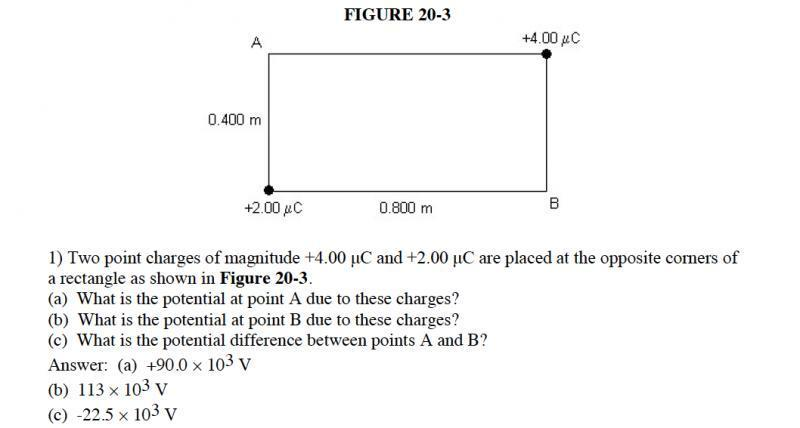# Find the potential difference in a rectangle

## Homework Statement

[/B]
Figure 20-3, referred to below, is 0.800m wide and 0.400m tall with "A" in the top left corner, "+4 microC" charge in the top right corner, "+2 microC" charge in the bottom left corner, and "B" in the bottom right corner.

Two point charges of magnitude +4.00 μC and +2.00 μC are placed at the opposite corners of a rectangle as shown in Figure 20-3.
(a) What is the potential at point A due to these charges?
(b) What is the potential at point B due to these charges?
(c) What is the potential difference between points A and B?

## Homework Equations

U=(kQq)/r where k=8.99E9 Nm^2/C^2

U=qV

V=(kq)/r

## The Attempt at a Solution

I honestly do not know where to begin this problem, other wise I wouldn't have posted it. I started by trying to plug values into "U=(kQq)/r" but quickly realized that would only help me if I knew the charge on points A and B.

This is what I had done before I realized I had no idea what I was doing:
U=(8.99E9*4*2)/0.89 (0.89 is the hypotenuse of the rectangle)

I was then going to plug that number into U=qV and solve for V, but I have no q for points A or B so I'm stuck.

Here is the Figure

#### Attachments

•Screen Shot 2014-10-15 at 10.41.41 PM.png
64.6 KB · Views: 2,440
nasu
Gold Member
Use the formula for potential. This is the quantity you have to calculate.
Calculate potential at A produced by each charge, separately. Then add the two potentials.

ehild
Homework Helper## Homework Equations

U=(kQq)/r where k=8.99E9 Nm^2/C^2
This is the potential energy of a charge q in the field of a a point charge Q. You need the potential, V, the potential energy of a unit positive charge. You wrote correctly that
U=qV

V=(kq)/r

## The Attempt at a Solution

I honestly do not know where to begin this problem, other wise I wouldn't have posted it. I started by trying to plug values into "U=(kQq)/r" but quickly realized that would only help me if I knew the charge on points A and B.

You need the potential V=kQ/r. that q in the formula for the potential energy is 1 C.

This is what I had done before I realized I had no idea what I was doing:
U=(8.99E9*4*2)/0.89 (0.89 is the hypotenuse of the rectangle)

I was then going to plug that number into U=qV and solve for V, but I have no q for points A or B so I'm stuck.

You do not need U. You need V. Recall, that the potential at distance r from a point charge Q is kQ/r.
The potential at A is the sum of the potentials from both the ##2 \mu C## charge and the
##4 \mu C## charge. Substitute the appropriate distances in the formula kQ/r. How far is A from both charges?

ehild